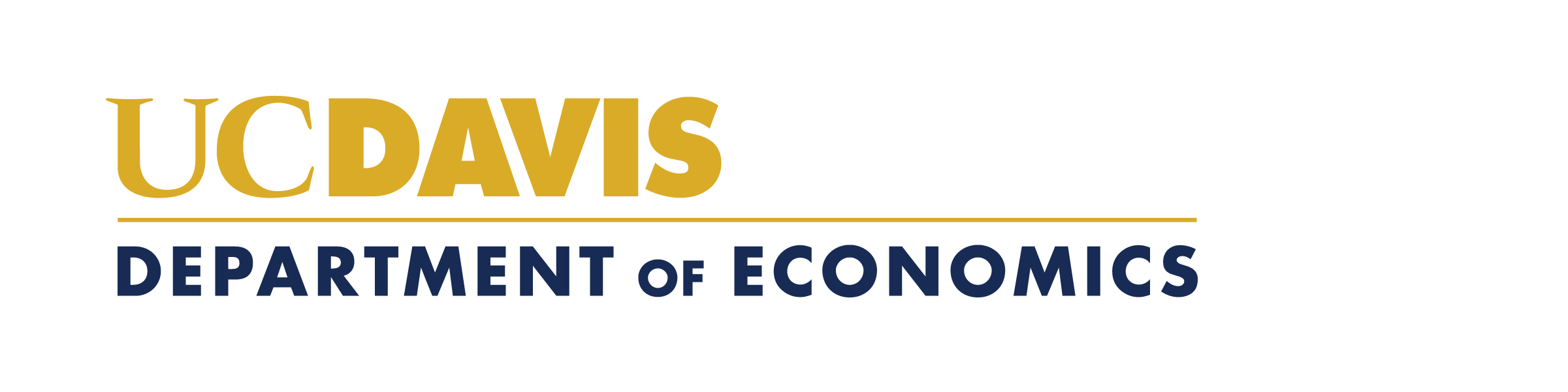A. Colin Cameron: Python for Regression

These notes are for data analysis using key Python modules, notably statsmodels for statistics and scikit-learn for machine learning. This requires little knowledge of how to program in Python (a low-level language). Instead one needs to know the commands to use the modules.
Rather than directly install Python it is convenient to install Anaconda which also automatically installs key modules.

Anaconda installs not only base Python plus many packages (collections of modules), including the key ones for econometrics.
- NumPy. Short for numbers in Python. Array data types needed for statistics and data analysis.
- pandas. Name derived from panel data. R type data frames and data analysis tools.
- matplotlib. Static and dynamic data visualizations.
- SciPy. Scientific library (optimization, integration, eigenvalue problems, random number generators, ...)
- statsmodels. Statistics using pandas dataframes (computations and models including standard regression models).
- scikit-learn (Sklearn). Machine learning and data mining using NumPy arrays.
- TensorFlow. Deep learning such as neural networks.
Commands for many of these modules have similar format to R commands.

Once Anaconda is installed you can run a Python program from within Anaconda.

For example, use the Spyder GUI interface (which is simlar to R Studio for R).
Or use the command shell.

Install and use Anaconda

Some Python coding tips

Python simple OLS regression example

Python Random Forest Example
Data for
Random Forest Example

You can also run Python within Stata.
This can be particularly useful for setting up data in Stata and then transferring data from Stata to Python.

Python within Stata random forest example

References useful for econometrics with Python

Kevin Sheppard, Introduction to Python for Econometrics, Statistics and Numerical Analysis: Fourth+ Edition
pdf at  https://www.kevinsheppard.com/teaching/python/notes/
Wes McKinney, Python for Data Analysis: data wrangling with pandas, NumPy, and Jupyter, Third edition.
html web version at https://wesmckinney.com/book/  and paperback at Amazon

Reference for machine learning with Python
Aurelien Geron, Hands-On Machine Learning with Scikit-Learn, Keras & Tensor Flow, Third Edition Amazon
Scikit-learn website has many examples.
Gareth James, Daniela Witten, Trevor Hastie, Robert Tibshirani, Jonathan Taylor, An Introduction to Statistical Learning: With Applications in Python, Springer, forthcoming. https://www.statlearning.com/A. Colin Cameron / UC-Davis Economics /  http://www.econ.ucdavis.edu/faculty/cameron